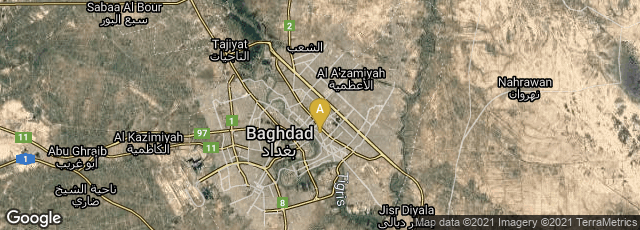# Ancient Babylonian Algorithms: The Earliest Programs

Circa 1800 to 1600 BCE

In 1972 computer scientist and mathematician Donald E. Knuth published "Ancient Babylonian Algorithms," in which he provided the first English translations of various cuneiform mathematical tablets, with commentary. The tablets he studied ranged in date from 1800-1600 BCE. As a reflection of how comparatively little prestige computer science had as an academic subject at the time, Knuth began his paper with the statement:

"One of the ways to help make computer science respectable is to show that is deeply rooted in history, not just a short-lived phenomenon. Therefore it is natural to turn to the earliest surviving documents which deal with computation, and to study how people approached the subject nearly 4000 years ago."

From his paper I offer a few selections:

" 'Babylonian Programming'

"The Babylonian mathematicians were not limited simply to the processes of addition, subtraction, multiplication, and division; they were adept at solving many types of algebraic equations. But they did not have an algebraic notation that is quite as transparent as ours; they represented each formula by a set-by-step list of rules for its evaluation, i.e. by an algorithm for computing that formula. In effect, they worked with a 'machine language' representation of formulas instead of a symbolic language.

"The flavor of Babylonian mathematics can best be appreciated by studying several examples. The translations below attempt to render the words of the original texts as faithfully as possible into good English, without extensive editorial interpretation. Several remarks have been added in parentheses, to explain some of the things that were originally unstated on the tables. All numbers are presented Babylonian-style, i.e. without exponents, so the reader is warned that he will have to supply an appropriate scale factor in his head; thus, it is necessary to remember that I might mean 60 and 15 might mean 1/4.

"The first example that we shall discuss is excerpted from an Old-Babylonian tablet which was originally about 5 x 8 x 1 inches in size. Half of it now appears in the British Museum, about one-fourth appears in the Staatliche Museen, Berlin, and the other fourth has apparently been lost or destroyed over the years....

##### This is the procedure.

" The first step here is to divide 27, 46, 40 by 3,20; this is reduced to muliplication by the reciprocal. The multiplication was done by referring to tables, probably by manipulating stones or sand in some manner and then writing down the answer. The square root was also computed by referring to tables, since we know that many tables of n vs. existed. Note that the rule for computing the values of x and y such that x - y =d and xy = p ≠ (d/2).

"The calculations described in Babylonian tablets are not merely the solutions to specific individual problems; they are actually general procedures for solving a whole class of problems. The numbers shown are merely included as an aid to exposition, in order to clarify the general method. This fact is clear because there are numerous instances where a particular case of the general method reduces to multiplying by 1; such a multiplication is explicity carried out, in order to abide by the general rules. Note also the stereotyped ending, 'This is the procedure,' which is commonly found at the end of each section on a table. Thus the Babylonian procedures are genuine algorithms, and we can commend the Babylonians for developing a nice way to explain an algorithm by example as the algorithm itself was being defined.... (pp. 672-73).

Knuth, Ancient Babylonian Algorithms, Communications of the ACM 15, no. 7 (July 1972) 671-77.